# Saxon Calculus: Trigonometry Chapter Exam

Exam Instructions:

Choose your answers to the questions and click 'Next' to see the next set of questions. You can skip questions if you would like and come back to them later with the yellow "Go To First Skipped Question" button. When you have completed the practice exam, a green submit button will appear. Click it to see your results. Good luck!

### Page 1

#### Question 3 3. Solve the following trigonometric equation for x.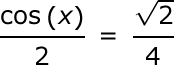### Page 3

#### Question 13 13. Solve the following equation for x.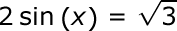#### Question 14 14. Simplify the following expression. Report the answer in degrees.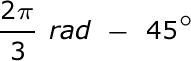### Page 4

#### Question 17 17. Perform the following calculation. Report the answer in radians.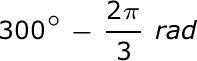#### Question 19 19. Convert the following measurement in radian to degrees.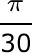### Page 5

#### Question 22 22. In the pictured triangle, what ratio represents the cosine of theta?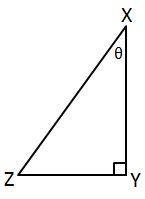### Page 6

#### Question 27 27. Solve the following equation for θ.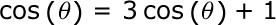#### Question 30 30. Solve for the value of x in the trigonometric equation below. (Use the domain -π/2 to π/2)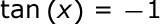#### Saxon Calculus: Trigonometry Chapter Exam Instructions

Choose your answers to the questions and click 'Next' to see the next set of questions. You can skip questions if you would like and come back to them later with the yellow "Go To First Skipped Question" button. When you have completed the practice exam, a green submit button will appear. Click it to see your results. Good luck!

Support